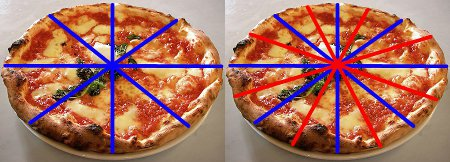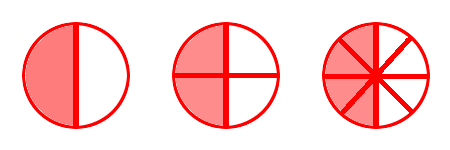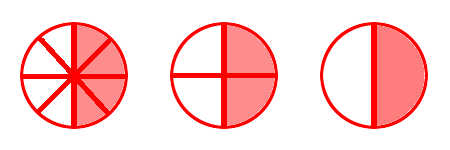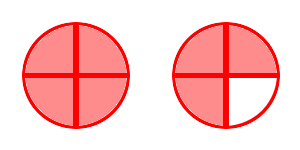# Equivalent FractionsIn the pizza on the left, what fraction is one slice of pizza? The answer is 1 part out of the 8 parts, one-eighth or 18.

In the pizza on the right, what fraction is two slices of pizza? The answer is 2 parts out of the 16 parts, two-sixteenths or 216.

These pizza servings are the same so 18 and 216 are equivalent fractions.

## Examples

Multiplying both numerator and denominator by the same number:1⁄2 = 2⁄4 = 4⁄8 To change 1⁄2 to 2⁄4, multiply both the numerator and the denominator by 2. To change 2⁄4 to 4⁄8, multiply both the numerator and the denominator by 2.2⁄3 = 4⁄6 = 8⁄12 To change 2⁄3 to 4⁄6, multiply both the numerator and the denominator by 2. To change 4⁄6 to 8⁄12, multiply both the numerator and the denominator by 2.

Dividing both numerator and denominator by the same number:4⁄8 = 2⁄4 = 1⁄2 To change 4⁄8 to 2⁄4, divide both the numerator and the denominator by 2. To change 2⁄4 to 1⁄2, divide both the numerator and the denominator by 2.8⁄12 = 4⁄6 = 2⁄3 To change 8⁄12 to 4⁄6, divide both the numerator and the denominator by 2. To change 4⁄6 to 2⁄3, divide both the numerator and the denominator by 2.

More examples of equivalent fractions:

34 = 912 (Multiplied by 3)

56 = 2024 (Multiplied by 4)

3036 = 56 (Divided by 6)

2030 = 23 (Divided by 10)

## Questions

What is the missing number, X?

Q1. 12 = X10
Q2. 13 = X12
Q3. 12 = 10X
Q4. 23 = X18
Q5. 25100 = X4

A1. 5
A2. 4
A3. 20
A4. 12
A5. 1

## Changing a Mixed Number to an Improper Fraction

A mixed number is a mixture of a whole number and a fraction. An example of a mixed number is 212 When a mixed number is converted to a fraction only, it is called an improper fraction. An example of an improper fraction is 5213⁄4 = 7⁄4 To change the mixed number of 13⁄4 to the improper fraction of 7⁄4,multiply the whole number 1 by the denominator 4,and then add on the numerator 3.

## Changing an Improper Fraction Back to a Mixed Number12⁄3 = 5⁄3 To change the mixed number of 12⁄3 to the improper fraction of 5⁄3,multiply the whole number 1 by the denominator 3,and then add on the numerator 2.